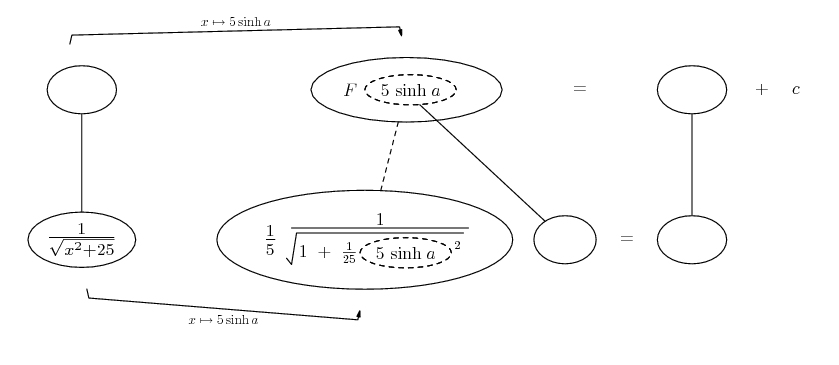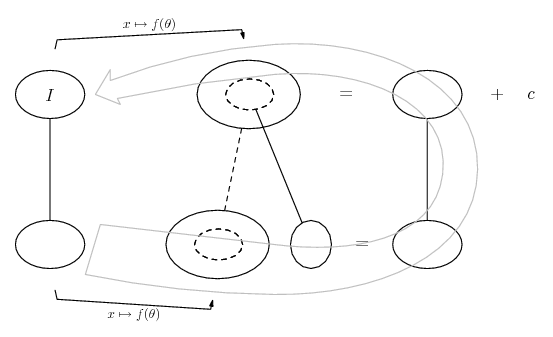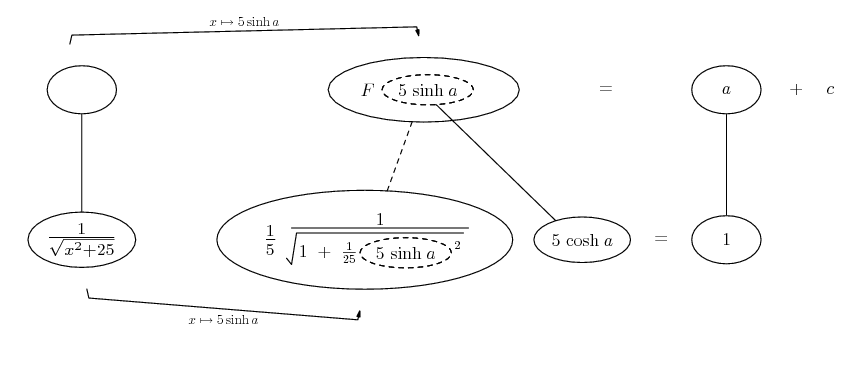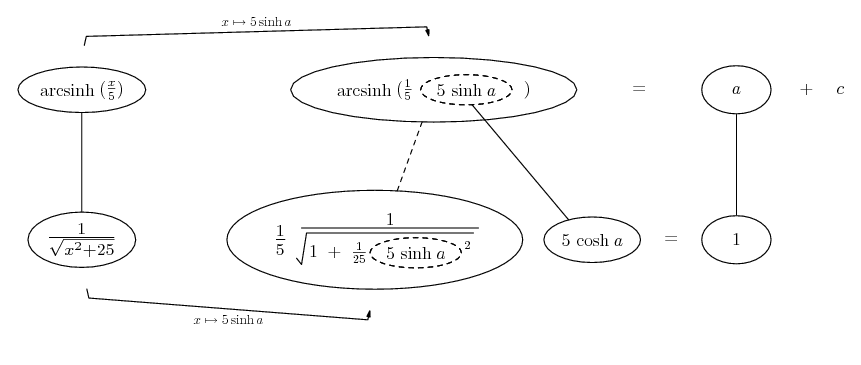# Thread: Intergration via trigonometric and hyperbolic substitutions

1. ## Intergration via trigonometric and hyperbolic substitutions

Intgrate(1/sqrt(x^2 + 25))

I started off by letting x=5sinh(a) then substituted in to get

intgral(5cosh(a)/sqrt(25sinh^2(a)+25))

=intgral(cosh(a)/cosh(a))

=x + c

This is not correct, so if anyone could explain where I went wrong and what I should do, that would be very very much appreciated.

Nic

2. Try x=5tan

3.Originally Posted by dwsmithTry x=5tan
That sub is fine, but you end up having to tackle sec.

$\displaystyle x \mapsto 5 \sinh a$ is good. Just in case a picture helps...... shows the sub.... is the chain rule. All straight continuous lines differentiate downwards (integrate up) with respect to the present variable (x or a), and the straight dashed line similarly but with respect to the dashed balloon expression (the inner function of the composite which is subject to the chain rule).

And the general drift is...Spoiler:Spoiler:Edit:

And (d'oh!) you weren't that wrong anyway - just back-sub. Still, hope you like the pic.
_________________________________________
Don't integrate - balloontegrate!

Balloon Calculus; standard integrals, derivatives and methods

Balloon Calculus Drawing with LaTeX and Asymptote!

hyperbolic, intergration, substitutions, trigonometric